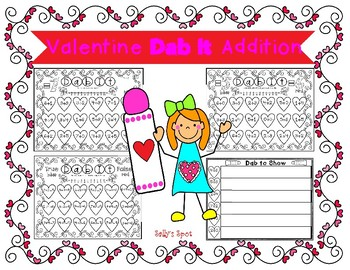# Valentine Dab It AdditionSubject
Grade Levels
Resource Type
Product Rating
4.0
1 Rating
File Type

PDF (Acrobat) Document File

Be sure that you have an application to open this file type before downloading and/or purchasing.

3 MB|14 pages
Share
Product Description

Valentine Dab It Addition

This is a great Valentine activity to help children learn the addition facts. Children love to use paint

dabbers. If you don’t have paint dabbers, the children can color the hearts instead. Paint dabbers are

well worth buying.

Dab It

The children can use the number line on each page to solve the equations if needed. They will dab all

the equations that equal the given answer/sum. They can write the sum on the heart.

7 Sheets Included:

=2, =3, =4

=5

=6

=7

=8

=9

=10

True or False (1 sheet)

The children will use a blue dabber to dab each

equation that is true and a red dabber to dab each

equation that is false. They can put the correct answer to the

false equations on the heart before dapping it.

Dab to Show

The children will use a different color dabber to dab each addend.

They will write the sum under the equation.

There are 5 of these sheets.

Total Pages
14 pages
Answer Key
N/A
Teaching Duration
N/A
Report this ResourceSign Up